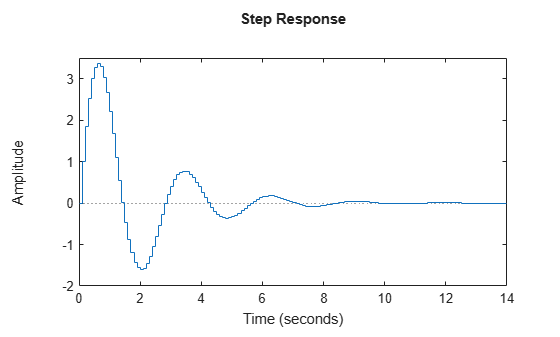# Creating Discrete-Time Models

This example shows how to create discrete-time linear models using the `tf`, `zpk`, `ss`, and `frd` commands.

### Specifying Discrete-Time Models

Control System Toolbox™ lets you create both continuous-time and discrete-time models. The syntax for creating discrete-time models is similar to that for continuous-time models, except that you must also provide a sample time (sampling interval in seconds).

For example, to specify the discrete-time transfer function:

`$H\left(z\right)=\frac{z-1}{{z}^{2}-1.85z+0.9}$`

with sampling period `Ts = 0.1 s`, type:

```num = [ 1 -1 ]; den = [ 1 -1.85 0.9 ]; H = tf(num,den,0.1)```
```H = z - 1 ------------------ z^2 - 1.85 z + 0.9 Sample time: 0.1 seconds Discrete-time transfer function. ```

or equivalently:

```z = tf('z',0.1); H = (z - 1) / (z^2 - 1.85*z + 0.9);```

Similarly, to specify the discrete-time state-space model:

`$x\left[k+1\right]=0.5x\left[k\right]+u\left[k\right]$`

`$y\left[k\right]=0.2x\left[k\right].$`

with sampling period `Ts = 0.1 s`, type:

`sys = ss(.5,1,.2,0,0.1);`

### Recognizing Discrete-Time Systems

There are several ways to determine if your LTI model is discrete:

• The display shows a nonzero sample time value

• `sys.Ts` or `get(sys,'Ts')` return a nonzero sample time value.

• `isdt(sys)` returns true.

For example, for the transfer function `H` specified above,

`H.Ts`
```ans = 0.1000 ```
`isdt(H)`
```ans = logical 1 ```

You can also spot discrete-time systems by looking for the following traits:

• Time response plots - Response curve has a staircase look owing to its sampled-data nature

• Bode plots - There is a vertical bar marking the Nyquist frequency (pi divided by the sample time).

The following plots show these characteristic traits:

`step(H)``bode(H), grid`